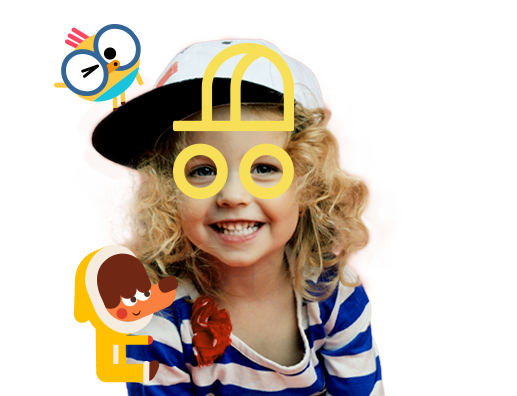[Image_Link]https://ct.pinterest.com/v3/?event=init&tid=2613246001233&pd[em]=&noscript=1" />

English for Kids. FREE playlearning™ content curated by the Lingokids educators team.

## English for kids

Free Playlearning™ content curated by the Lingokids educators team.# Doubles Song 🔢 Music for Math Skills

## “Doubles” Song Lyrics

Doubles, doubles

Doubles, doubles

Doubles, doubles

Doubles

Look how easy it can be.

Sing with me!

1+1=2

2+2=4

3+3=6

4+4=8

5+5=10

Now let’s add the bigger ones.

6+6=12

7+7=14

8+8=16

9+9=18

10+10=20

Now you know how to add!

Doubles, doubles

Doubles, doubles

Doubles, doubles

Doubles

Look how easy it can be.

Sing with me!

1+1=2

2+2=4

3+3=6

4+4=8

5+5=10

Now let’s add the bigger ones.

6+6=12

7+7=14

8+8=16

9+9=18

10+10=20

Now you know how to add!